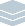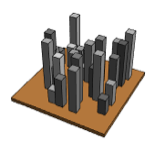Wolfram Archive
Wolfram Programming Lab is a legacy product.
All the same functionality and features, including access to Programming Lab Explorations, are available with Wolfram|One.
Start programming now. »
Try it now »
(no sign-in required)# Random Cityscapes

Create a random arrangement of skyscrapers.

Run the code to place 30 random squares in a size-10 region. Try numbers other than 30:

This draws a default rectangle, which is a square:

Graphics[Rectangle[]]

Add PlotRange{{0,10},{0,10}} to make the drawing area extend from 0 to 10 in both directions, and AxesTrue to turn on axes so you can see the coordinate values:

Graphics[Rectangle[], PlotRange -> {{0, 10}, {0, 10}}, Axes -> True]

You can draw a square in a random position using RandomReal.

This gives a random number between 0 and 10. Each time you run the code, you get a different number:

RandomReal

This gives a pair of random numbers between 0 and 10. Each time you run the code, you get a different pair:

RandomReal[10, 2]

Use RandomReal to draw a square in a random position. Each time you run the code, the square is in a different place:

Graphics[Rectangle[RandomReal[10, 2]], PlotRange -> {{0, 10}, {0, 10}}, Axes -> True]

Table makes lists of things. This uses Table to make a list of 5 random numbers:

Table[RandomReal, {5}]

This uses Table to draw 30 squares in random positions:

Graphics[Table[Rectangle[RandomReal[10, 2]], {30}], PlotRange -> {{0, 10}, {0, 10}}, Axes -> True]

Turn off the axes by removing AxesTrue, and remove PlotRange{{0,10},{0,10}} too. Without an explicit PlotRange value, the plot range is calculated automatically to be just large enough to contain the contents of the graphic:

Graphics[Table[Rectangle[RandomReal[10, 2]], {30}]]

Graphics[Table[Rectangle[RandomReal[10, 2]], {30}]]

Use 3D cuboids instead of 2D squares. Try numbers other than 30:

This draws a square at {0,0}:

Graphics[Rectangle[{0, 0}]]

This draws a cube at {0,0,0}. The expression for drawing a cube is like the one for drawing a square, but Graphics is replaced by Graphics3D, Rectangle by Cuboid and the two-dimensional coordinate {0,0} by the three-dimensional coordinate {0,0,0}:

Graphics3D[Cuboid[{0, 0, 0}]]

You can draw cubes in random positions similar to the way you drew squares in random positions. In addition to replacing Graphics with Graphics3D and Rectangle with Cuboid, you need to replace RandomReal[10,2] with RandomReal[10,3] so that the coordinate values are three-dimensional:

Graphics3D[Table[Cuboid[RandomReal[10, 3]], {30}]]

To draw cubes in random positions but with the same vertical coordinate, make a two-dimensional coordinate with RandomReal[10,2] and append zero to it:

Append[RandomReal[10, 2], 0]

Use that code to make random cubes that all lie in the same vertical position:

Graphics3D[Table[Cuboid[Append[RandomReal[10, 2], 0]], {30}]]

Graphics3D[Table[Cuboid[Append[RandomReal[10, 2], 0]], {30}]]

Pick random heights between 2 and 8. Try ranges of heights other than {2,8}for example, {1,2}:

This draws a box with corners at coordinates {5,5,0} and {6,6,7}:

Graphics3D[ Cuboid[{5, 5, 0}, {6, 6, 7}], PlotRange -> {{0, 11}, {0, 11}, {0, 11}}]

Another way to write the same thing is to separate the position of the box{5,5,0}from the size of the box{1,1,7}. The first corner coordinate is given by the position, and the second by the position + size:

Graphics3D[ Cuboid[{5, 5, 0}, {5, 5, 0} + {1, 1, 7}], PlotRange -> {{0, 11}, {0, 11}, {0, 11}}]

A better way to write the same thing is to use With so that if you want to change the boxs position, you only have to change the code in one place:

Graphics3D[ With[{pos = {5, 5, 0}}, Cuboid[pos, pos + {1, 1, 7}]], PlotRange -> {{0, 11}, {0, 11}, {0, 11}}]

Now its easy to change the code to draw a height 7 box at a random position. Just replace pos={5,5,0} with pos=Append[RandomReal[10,2],0]. Run this code repeatedly to see the box move around:

Graphics3D[ With[{pos = Append[RandomReal[10, 2], 0]}, Cuboid[pos, pos + {1, 1, 7}]], PlotRange -> {{0, 11}, {0, 11}, {0, 11}}]

Make the height vary between 2 and 8 by replacing 7 with RandomReal[{2,8}]:

Graphics3D[ With[{pos = Append[RandomReal[10, 2], 0]}, Cuboid[pos, pos + {1, 1, RandomReal[{2, 8}]}]], PlotRange -> {{0, 11}, {0, 11}, {0, 11}}]

Use Table to draw 30 random boxes and remove PlotRange{{0,11},{0,11},{0,11}} so that the output is just big enough to contain the boxes:

Graphics3D[ Table[ With[{pos = Append[RandomReal[10, 2], 0]}, Cuboid[pos, pos + {1, 1, RandomReal[{2, 8}]}]], {30}] ]

Graphics3D[ Table[With[{pos = Append[RandomReal[10, 2], 0]}, Cuboid[pos, pos + {1, 1, RandomReal[{2, 8}]}]], {30}]]

Use neutral lighting (and remove the box):

Use BoxedFalse to draw the graphic without a box around it:

Graphics3D[ Table[With[{pos = Append[RandomReal[10, 2], 0]}, Cuboid[pos, pos + {1, 1, RandomReal[{2, 8}]}]], {30}], Boxed -> False]

Add Lighting"Neutral" to get white lighting:

Graphics3D[ Table[With[{pos = Append[RandomReal[10, 2], 0]}, Cuboid[pos, pos + {1, 1, RandomReal[{2, 8}]}]], {30}], Boxed -> False, Lighting -> "Neutral"]

Graphics3D[ Table[With[{pos = Append[RandomReal[10, 2], 0]}, Cuboid[pos, pos + {1, 1, RandomReal[{2, 8}]}]], {30}], Boxed -> False, Lighting -> "Neutral"]

Use random gray levels between .3 and .7 for the skyscrapers. Try ranges of gray levels other than {.3,.7}for example, {0,1}:

This draws a white box:

Graphics3D[ Cuboid[], Boxed -> False, Lighting -> "Neutral"]

This draws a 50% gray box:

Graphics3D[ {GrayLevel[.5], Cuboid[]}, Boxed -> False, Lighting -> "Neutral"]

This draws a box with a random gray color between 30% and 70%. Each time you run the code, you get a different gray:

Graphics3D[ {GrayLevel[RandomReal[{.3, .7}]], Cuboid[]}, Boxed -> False, Lighting -> "Neutral"]

Add GrayLevel[RandomReal[{.3,.7}]] to the skyscraper code to get randomly colored buildings:

Graphics3D[ Table[With[{pos = Append[RandomReal[10, 2], 0]}, {GrayLevel[ RandomReal[{.3, .7}]], Cuboid[pos, pos + {1, 1, RandomReal[{2, 8}]}]}], {30}], Boxed -> False, Lighting -> "Neutral"]

Graphics3D[ Table[With[{pos = Append[RandomReal[10, 2], 0]}, {GrayLevel[ RandomReal[{.3, .7}]], Cuboid[pos, pos + {1, 1, RandomReal[{2, 8}]}]}], {30}], Boxed -> False, Lighting -> "Neutral"]

Add ground for the skyscrapers to stand on. Try colors other than Brownfor example, Pink or Darker[Green]:

Add another Cuboid to represent the ground. The skyscrapers are positioned between 0 and 10, and they are 1 unit wide and deep, so their footprints will be anywhere between 0 to 11 in the x and y directions. Add a 1-unit margin around the edges for a range of -1 to 12.

The bottoms of the skyscrapers are at z coordinate 0. Make the ground cuboid .5 units thick by making it extend downward to -.5. That gives Cuboid[{{-1,-1,-.5},{12,12,0}}] for the ground:

Graphics3D[{ Cuboid[{-1, -1, -.5}, {12, 12, 0}], Table[With[{pos = Append[RandomReal[10, 2], 0]}, {GrayLevel[ RandomReal[{.3, .7}]], Cuboid[pos, pos + {1, 1, RandomReal[{2, 8}]}]}], {30}] }, Boxed -> False, Lighting -> "Neutral"]

Color the ground Brown:

Graphics3D[{ Brown, Cuboid[{-1, -1, -.5}, {12, 12, 0}], Table[With[{pos = Append[RandomReal[10, 2], 0]}, {GrayLevel[ RandomReal[{.3, .7}]], Cuboid[pos, pos + {1, 1, RandomReal[{2, 8}]}]}], {30}] }, Boxed -> False, Lighting -> "Neutral"]

Graphics3D[{ Brown, Cuboid[{-1, -1, -.5}, {12, 12, 0}], Table[With[{pos = Append[RandomReal[10, 2], 0]}, {GrayLevel[ RandomReal[{.3, .7}]], Cuboid[pos, pos + {1, 1, RandomReal[{2, 8}]}]}], {30}] }, Boxed -> False, Lighting -> "Neutral"]

Interactively fill in skyscrapers. Drag the slider to fill in the landscape:

This is the code to make a landscape with 30 skyscrapers:

Graphics3D[{ Brown, Cuboid[{-1, -1, -.5}, {12, 12, 0}], Table[With[{pos = Append[RandomReal[10, 2], 0]}, {GrayLevel[ RandomReal[{.3, .7}]], Cuboid[pos, pos + {1, 1, RandomReal[{2, 8}]}]}], {30}] }, Boxed -> False, Lighting -> "Neutral"]

You can make the landscape interactive with Manipulate.

Manipulate attaches interactive controls to things like this expression for 3 squared:

3^2

Make that expression interactive by wrapping it with Manipulate[...], replacing the 3 with n and specifying that n goes from 0 to 30 in steps of 1. Drag the slider to see the squares of various numbers:

Manipulate[n^2, {n, 0, 30, 1}]

You can do the same thing with the skyscraper expression to make it interactive. Wrap it with Manipulate[...], replace the 30 with n and specify that n goes from 0 to 30 in steps of 1. Drag the slider to see the number of buildings change:

Manipulate[ Graphics3D[{ Brown, Cuboid[{-1, -1, -.5}, {12, 12, 0}], Table[With[{pos = Append[RandomReal[10, 2], 0]}, {GrayLevel[ RandomReal[{.3, .7}]], Cuboid[pos, pos + {1, 1, RandomReal[{2, 8}]}]}], {n}] }, Boxed -> False, Lighting -> "Neutral"], {n, 0, 30, 1} ]

The buildings jump around because RandomReal gives different numbers each time it is used. You can make them stay put by adding SeedRandom to the code:

Manipulate[ SeedRandom; Graphics3D[{ Brown, Cuboid[{-1, -1, -.5}, {12, 12, 0}], Table[With[{pos = Append[RandomReal[10, 2], 0]}, {GrayLevel[ RandomReal[{.3, .7}]], Cuboid[pos, pos + {1, 1, RandomReal[{2, 8}]}]}], {n}] }, Boxed -> False, Lighting -> "Neutral"], {n, 0, 30, 1} ]

Heres why SeedRandom keeps the buildings from jumping around.

RandomReal gives a different number each time it is used, so each time a new landscape is drawn, the buildings are in different positions.

SeedRandom resets the random number generator so that subsequent uses of RandomReal give the same sequence of random numbers.

This list of random numbers changes each time you run the code:

Table[RandomReal[], {5}]

This list of random numbers is always the same because the random number generator is reset with SeedRandom:

SeedRandom; Table[RandomReal[], {5}]

You can give different numbers to SeedRandom to reset the random number generator to different places.

Manipulate[ SeedRandom; Graphics3D[{ Brown, Cuboid[{-1, -1, -.5}, {12, 12, 0}], Table[With[{pos = Append[RandomReal[10, 2], 0]}, {GrayLevel[ RandomReal[{.3, .7}]], Cuboid[pos, pos + {1, 1, RandomReal[{2, 8}]}]}], {n}] }, Boxed -> False, Lighting -> "Neutral"], {n, 0, 30, 1} ]

Share Itmake an interactive cityscapes website:

Deploy the Manipulate to the Wolfram Cloud, where anyone with a browser can use it:

CloudDeploy[ Manipulate[ SeedRandom; Graphics3D[{ Brown, Cuboid[{-1, -1, -.5}, {12, 12, 0}], Table[ With[{pos = Append[RandomReal[10, 2], 0]}, {GrayLevel[ RandomReal[{.3, .7}]], Cuboid[pos, pos + {1, 1, RandomReal[{2, 8}]}]}], {n}] }, Boxed -> False, Lighting -> "Neutral"], {n, 0, 30, 1} ], Permissions -> "Public" ]

Click the link in the output to visit the site.